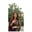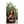Trusted answers to developer questions
Trusted Answers to Developer Questions

Related Tags

community creator
steiner
tree
graph

# What is the Steiner Tree Problem?Arooj Fatima

Grokking Modern System Design Interview for Engineers & Managers

Ace your System Design Interview and take your career to the next level. Learn to handle the design of applications like Netflix, Quora, Facebook, Uber, and many more in a 45-min interview. Learn the RESHADED framework for architecting web-scale applications by determining requirements, constraints, and assumptions before diving into a step-by-step design process.

Named after Jakob Steiner, the Steiner Tree Problem covers a class of problems in combinatorial optimization.

Steiner Tree Problems require an optimal interconnect for a given set of objects and a predefined objective function. The Steiner Tree Problem in graphs is one of the variants of this problem.

## What is a Steiner Tree?

A Steiner Tree is an undirected, weighted graph with a minimum-weight tree that connects a selected set of vertices, called terminals.

Steiner vertices:

The tree may include non-terminals, which are called Steiner points or Steiner vertices.

## Applications of the Steiner Tree

Some of the areas where a Steiner Tree is used:

• VLSI designVery-large-scale integration
• network routing
• wireless communications
• computational biology

## Problem

Given:

• an undirectededges that do not have a direction graph
• non-negative edge weights
• a subset of vertices/ terminals

The Steiner Tree in a graph is a Minimum Spanning Treea tree that minimizes the lengths (or “weights”) of the edges of the tree - “T” of minimum weight that contains all given terminals, including additional vertices.

## How is it found?

Start with a subtreetree which is a child of a node that consists of one given terminal vertex. In this case, the starting vertex is B. A subtree does not span all terminals.

Select a terminal (x) closest to a vertex in the subtree, but not within the subtree itself.

Add the shortest path that connects x to the subtree.

There are approximate algorithms to solve the same problem. The Steiner Tree problem is NP-Hardnondeterministic polynomial time; there are no polynomial-time solutions that always give optimal cost.

## Solution

Follow the steps below to find the solution in subtree T.

Step 1: Add terminal b to subtree T.

adding b to subtree

Step 2: Select a terminal that is closest to a vertex in T, but not in T itself.

Now the vertices connected to b are:

• a (4)
• c (6)
• d (5)

Choose the terminal with the minimum weight i.e. vertex a.

Step 3: Repeat step 2 by following Dijkstra’s Algorithm until all vertices are traversed.

Step 4: The final subtree will be as follows.

Formula for Dijkstra’s Algorithm

dx(y) → cost of least-cost path from x to y

then,

dx(y) = minvmin taken over all neighbors v of x { c(x,v)cost to neighbor v+ dv(y)cost from neighbor v to destination y

## The cost of a Steiner Tree

To find the costthe sum of cost/weight on its edges of a Steiner Tree, add the weights of the updated tree with the shortest path.

The cost of Steiner Tree is:

path: b-a-d-c-e-f-g

cost: 0+4+5+6+7+7+10

Cost = 39

RELATED TAGS

community creator
steiner
tree
graph

CONTRIBUTORArooj Fatima

Grokking Modern System Design Interview for Engineers & Managers

Ace your System Design Interview and take your career to the next level. Learn to handle the design of applications like Netflix, Quora, Facebook, Uber, and many more in a 45-min interview. Learn the RESHADED framework for architecting web-scale applications by determining requirements, constraints, and assumptions before diving into a step-by-step design process.

Keep Exploring

Learn in-demand tech skills in half the time